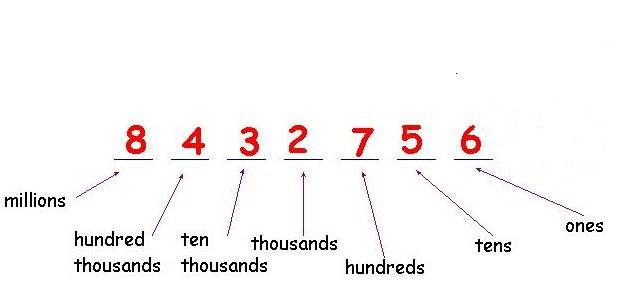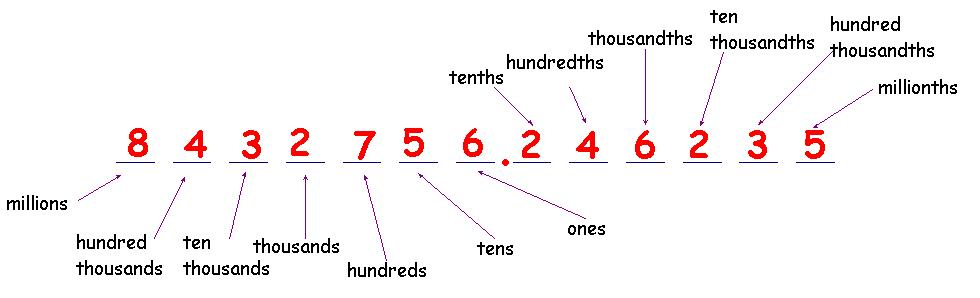HELP MATHS PLACE VALUE Return The position (or place) of a digit in a number determines the actual value the digit represents. This picture shows the place value for different positions:The number 8 432 756 has a 8 in the millions place, a 4 in the hundred thousands place, a 3 in the ten thousands place, a 2 in the thousands place, a 7 in the hundreds place, a 5 in the tens place and a 6 in the ones place. Expanded Form The expanded form of a number is the sum of its place values: 8 432 756 = 8 000 000  + 400 000 + 30 000 + 2 000 + 700 + 50 + 6 DECIMAL PLACE VALUE In the same way, in the decimal number system ,the value of a digit depends on where it is placed: